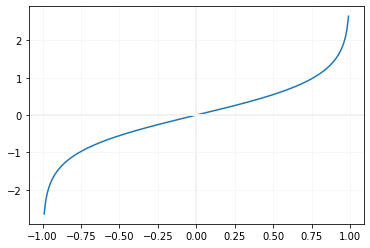# atanh()

atanh(x) returns inverse hyperbolic tangent of x , the input number x is in radian.
``````import math
print(math.atanh(-0.9))  # -1.4722194895832204
print(math.atanh(0))     # 0.0
print(math.atanh(0.9))   # 1.4722194895832204``````
Note that all the inputs are in radian.
less than or equal to -1 or more than equal to 1 , we will get ValueError
``````import math
print(math.atanh(1.0))  ``````
Above code will generate error.

## Inputs in degree

We can convert radian value to degree and use the same
``````import math
in_degree = 50
print(math.atanh(in_redian)) # 1.344146641636003``````
Output
``1.344146641636003``

## Drawing graph of atanh()

We will use Matplotlib to generate graph of atanh``````import matplotlib.pyplot as plt
x=[]
y=[]
i=-0.99
while (i<1):
x.append(i)
y.append(math.atanh(i))
i=i+0.01
plt.plot(x,y)
plt.axvline(x=0.00,linewidth=2, color='#f1f1f1')
plt.axhline(y=0.00,linewidth=2, color='#f1f1f1')
plt.grid(linestyle='-',
linewidth=0.5,color='#f1f1f1')
plt.show()``````

Subscribe to our YouTube Channel here

## Subscribe

* indicates required
Subscribe to plus2netplus2net.com